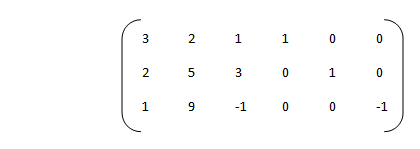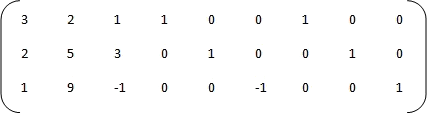# The 2-Phase Method

### Basic concepts and Principles

We start from a linear programming problem as
Min Ctx
Subject to
Ax ≤ b
x ≥ 0
where, as ever
A∈ Mmxn, rank(A)=m, b∈Rm, C∈Rn

Note at first, thar this ploblem is not written in standard form (see section , The simplex Algorithm)

If you want to see a two phase method complete example click here.

We have seen at section Simplex Pivot element how to pass from a Linear programming problem to it standard form by slack variables use.

The problem is, as we have seen, to find an identity mxm submatrix of A for starting simplex algorithm, wich can be not easy.

For this, we will apply the called two-phase method consisting of the following. Essentialy whose steps are in resume:

### Two-phase method overview

Phase 1

1) We look for an identity submatrix of dimension mxn within the total matrix A, once the slack variables are added, it will be necessary as much m artificial new artificial variables to start the algorithm.

In spite of being a reduntant, we will introduce m new artificial variablesm so we add a complete matrix mxm to the matrix A. With this we do not alter the set of extreme points of the original problem, and at the most we add any new step to the first phase. What we gain is to remove the complexity of distinguishing between original, slack and artificial variables at the time of going from the first to the second phase.

Most clear: In theory, less than m columas should be added since the original matrix together with the slack variables usually provide enough columns of the identity sub-Matrix. What we do here is that if we have to add a single artificial variable, then we add the full m. Why? Because we think that although it adds some more dimensions to the problem, it reduces its practicle complexity.

2) Change the original objective function for one that has all zeros except for last m components having the value 1 (ie, vector C = (0, .., n-times ..., 0, 1, .., m-times ..., 1)
3) Start the algorithm with this problem until to take one of these cases:

3a) We reached the optimal solution if zero: this means that artificial variables have left of basis, in this case we can move to phase 2 of the method.

3b) If we reached the optimal solution finite non-zero: The original problem has no solution.

Phase 2

Eliminate artificial variables, and continue algorithm, by:
1) Take the original objective function C

2) Take the m-firsts columns of the A matrix

3) Continue the algorithm with these changes until reach one of the 4 possible outputs of the problem.

The idea of phase 1 is to remove the artificial variables from the basis and get the trivial solution for the exthended problem. At this case, we can to pass to phase-two by eliminating artificial vars.

We will see in this section an example of the two phase method and how to handle artificial and slack variables. An even more complete is here.

### Extended Theory

We saw that every linear programming problem can be transformed into a standard form, for example if we have

Max(2x1 + 3x2 + 4x3)
Subject to
3x1 + 2x2 + x3 ≤ 10
2x1 + 5x2 + 3x3 ≤ 15
x1 + 9x2 - x3 ≥ 4
x1, x2, x3 ≥ 0

We can transform as follows
1) Change the sign of the objective function for a minimization problem

Min(-2x1 - 3x2 - 4x3)

2) Change the sign of the last of the restrictions:

3x1 + 2x2 + x3 ≤ 10
2x1 + 5x2 + 3x3 ≤ 15
-x1 - 9x2 + x3 ≤ -4

3) Introduce slack variables to remove inequalities and transform them into equalities:

3x1 + 2x2 + x3 + x4 = 10
2x1 + 5x2 + 3x3 + x5 = 15
-x1 - 9x2 + x3 + x6 = -4
x1, x2, x3, x4, x5, x6 ≥ 0

4) And the last, change of sign to the last of restrictions

3x1 + 2x2 + x3 + x4 = 10
2x1 + 5x2 + 3x3 + x5 = 15
x1 + 9x2 - x3 - x6 = 4
x1, x2, x3, x4, x5, x6 ≥ 0

We already have the standard formulation problem, what was a problem in 3 dimensions, we have become one of 6 dimensions, which is not too important, for the final calculations will be the computer who make them for us.
With these changes, the matrix A is is as followsAs we can see, we have not yet an identity submatrix to start the algorithm.
The solution is to apply the method of the two phases, which consists of the following:

Phase 1

1) We add a dummy variable for each of our restrictions, which will have no impact on them

3x1 + 2x2 + x3 + x4 + x7 = 10
2x1 + 5x2 + 3x3 + x5 + x8 = 15
x1 + 9x2 - x3 - x6 + x9= 4
x1, x2, x3, x4, x5, x6, x7, x8, x9 ≥ 0

The matrix A is nowNote that now we have an identity submatrix to start the simplex algorithm iterations.
2) We start the algorithm, but we as a vector of zero costs for all components, except those that correspond to dummy variables, ie we take the objective function so:

Min (x7 + x8 + x9)

So, the cost vector is

Ct = (0,0,0,0,0,0,1,1,1)

The idea is to obtain a solution with all artificial variables =0, ie, we want to find a solution S0 such us

x7, x8, x9 = 0

This will make the artificial variables out of the base.
Any other solution we obtain (finite or infinite) at the end of the first phase, will make our problem has no solution.

Phase 2

If we have founded S0, the solution at the end of the first phase, we eliminate the artificial variables and change the objective function for the original, in our example we again take

Min(-2x1 - 3x2 - 4x3)

As the objetive function, therfore the cost vector is now:

Ct = (-2,-3,-4,0,0,0)

Now we apply the algorithm
It should be noted that if we get optimal solution for variables that are not original (basic variables) simply have to remove them from our solution, ie, we make zero.
For this example we have a finite optimal solution obtained numerically:

x1 = 0.0
x2 = 0.84375
x3 = 3.59375000000000

What makes our maximization

Z = 16.90625

Execute this sample here with our apps!!

In practice the number of iterations of the simplex algorithm is between 3m / 2 and 3m, which is not bad if as we know for theory the number of extreme points is
(nm) = n!/m!(n-m)!
We apologize for not having a better notation for combinatorial numbers.

# Was useful? want add anything?

Post here

### Post from other users

Post here